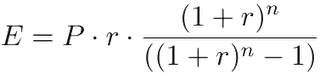InvestorQ : how are personal loan rates calculated ?# how are personal loan rates calculated ?Answer2 years ago
Followwhere E is emi , P is principal loan amount , r is rate of interest calculated on monthly basis. (i.e., r = Rate of Annual interest/12/100. If rate of interest is 10.5% per annum, then r = 10.5/12/100=0.00875) , n is loan term / tenure / duration in number of months.
2 Views